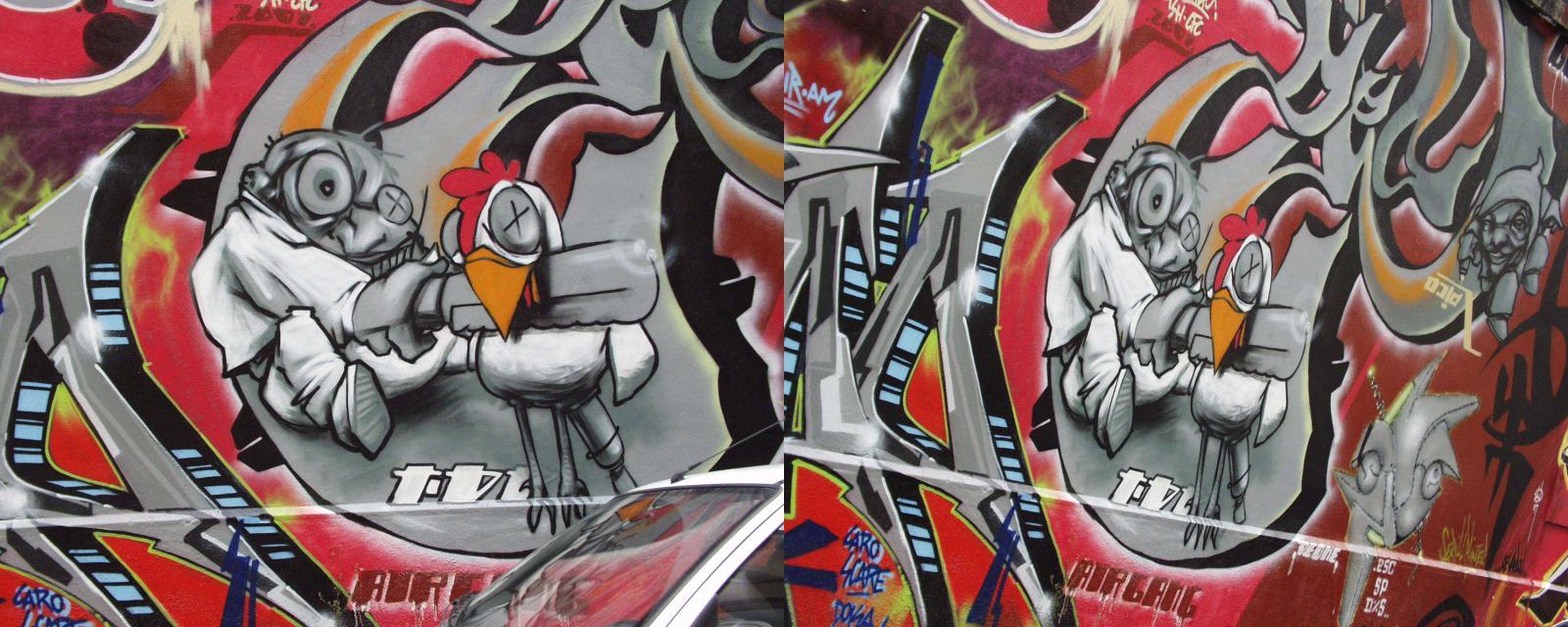OpenCV  3.4.0 Open Source Computer Vision
AKAZE local features matching

## Introduction

In this tutorial we will learn how to use AKAZE  local features to detect and match keypoints on two images. We will find keypoints on a pair of images with given homography matrix, match them and count the

number of inliers (i. e. matches that fit in the given homography).

You can find expanded version of this example here: https://github.com/pablofdezalc/test_kaze_akaze_opencv

## Data

We are going to use images 1 and 3 from Graffity sequence of Oxford dataset.Homography is given by a 3 by 3 matrix:

7.6285898e-01 -2.9922929e-01 2.2567123e+02
3.3443473e-01 1.0143901e+00 -7.6999973e+01
3.4663091e-04 -1.4364524e-05 1.0000000e+00

You can find the images (graf1.png, graf3.png) and homography (H1to3p.xml) in opencv/samples/cpp.

### Source Code

#include <iostream>
using namespace std;
using namespace cv;
const float inlier_threshold = 2.5f; // Distance threshold to identify inliers
const float nn_match_ratio = 0.8f; // Nearest neighbor matching ratio
int main(int argc, char* argv[])
{
CommandLineParser parser(argc, argv,
"{@img1 | ../data/graf1.png | input image 1}"
"{@img2 | ../data/graf3.png | input image 2}"
"{@homography | ../data/H1to3p.xml | homography matrix}");
Mat homography;
fs.getFirstTopLevelNode() >> homography;
vector<KeyPoint> kpts1, kpts2;
Mat desc1, desc2;
Ptr<AKAZE> akaze = AKAZE::create();
akaze->detectAndCompute(img1, noArray(), kpts1, desc1);
akaze->detectAndCompute(img2, noArray(), kpts2, desc2);
vector< vector<DMatch> > nn_matches;
matcher.knnMatch(desc1, desc2, nn_matches, 2);
vector<KeyPoint> matched1, matched2, inliers1, inliers2;
vector<DMatch> good_matches;
for(size_t i = 0; i < nn_matches.size(); i++) {
DMatch first = nn_matches[i];
float dist1 = nn_matches[i].distance;
float dist2 = nn_matches[i].distance;
if(dist1 < nn_match_ratio * dist2) {
matched1.push_back(kpts1[first.queryIdx]);
matched2.push_back(kpts2[first.trainIdx]);
}
}
for(unsigned i = 0; i < matched1.size(); i++) {
Mat col = Mat::ones(3, 1, CV_64F);
col.at<double>(0) = matched1[i].pt.x;
col.at<double>(1) = matched1[i].pt.y;
col = homography * col;
col /= col.at<double>(2);
double dist = sqrt( pow(col.at<double>(0) - matched2[i].pt.x, 2) +
pow(col.at<double>(1) - matched2[i].pt.y, 2));
if(dist < inlier_threshold) {
int new_i = static_cast<int>(inliers1.size());
inliers1.push_back(matched1[i]);
inliers2.push_back(matched2[i]);
good_matches.push_back(DMatch(new_i, new_i, 0));
}
}
Mat res;
drawMatches(img1, inliers1, img2, inliers2, good_matches, res);
imwrite("akaze_result.png", res);
double inlier_ratio = inliers1.size() * 1.0 / matched1.size();
cout << "A-KAZE Matching Results" << endl;
cout << "*******************************" << endl;
cout << "# Keypoints 1: \t" << kpts1.size() << endl;
cout << "# Keypoints 2: \t" << kpts2.size() << endl;
cout << "# Matches: \t" << matched1.size() << endl;
cout << "# Inliers: \t" << inliers1.size() << endl;
cout << "# Inliers Ratio: \t" << inlier_ratio << endl;
cout << endl;
imshow("result", res);
return 0;
}

### Explanation

Mat homography;
fs.getFirstTopLevelNode() >> homography;
We are loading grayscale images here. Homography is stored in the xml created with FileStorage.
2. Detect keypoints and compute descriptors using AKAZE
vector<KeyPoint> kpts1, kpts2;
Mat desc1, desc2;
AKAZE akaze;
akaze(img1, noArray(), kpts1, desc1);
akaze(img2, noArray(), kpts2, desc2);
We create AKAZE object and use it's operator() functionality. Since we don't need the mask parameter, noArray() is used.
3. Use brute-force matcher to find 2-nn matches
BFMatcher matcher(NORM_HAMMING);
vector< vector<DMatch> > nn_matches;
matcher.knnMatch(desc1, desc2, nn_matches, 2);
We use Hamming distance, because AKAZE uses binary descriptor by default.
4. Use 2-nn matches to find correct keypoint matches
for(size_t i = 0; i < nn_matches.size(); i++) {
DMatch first = nn_matches[i];
float dist1 = nn_matches[i].distance;
float dist2 = nn_matches[i].distance;
if(dist1 < nn_match_ratio * dist2) {
matched1.push_back(kpts1[first.queryIdx]);
matched2.push_back(kpts2[first.trainIdx]);
}
}
If the closest match is ratio closer than the second closest one, then the match is correct.
5. Check if our matches fit in the homography model

for(int i = 0; i < matched1.size(); i++) {
Mat col = Mat::ones(3, 1, CV_64F);
col.at<double>(0) = matched1[i].pt.x;
col.at<double>(1) = matched1[i].pt.y;
col = homography * col;
col /= col.at<double>(2);
float dist = sqrt( pow(col.at<double>(0) - matched2[i].pt.x, 2) +
pow(col.at<double>(1) - matched2[i].pt.y, 2));
if(dist < inlier_threshold) {
int new_i = inliers1.size();
inliers1.push_back(matched1[i]);
inliers2.push_back(matched2[i]);
good_matches.push_back(DMatch(new_i, new_i, 0));
}
}

If the distance from first keypoint's projection to the second keypoint is less than threshold, then it it fits in the homography.

We create a new set of matches for the inliers, because it is required by the drawing function.

6. Output results
Mat res;
drawMatches(img1, inliers1, img2, inliers2, good_matches, res);
imwrite("res.png", res);
...
Here we save the resulting image and print some statistics.

## Found matches## A-KAZE Matching Results

Keypoints 1: 2943
Keypoints 2: 3511
Matches: 447
Inliers: 308
Inlier Ratio: 0.689038}